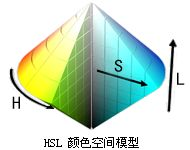# HSLtoRGB

This function is used to convert the HSL color to RGB color.

``````COLORREF HSLtoRGB(
float H,
float S,
float L
);``````

## Parameters

### H

Hue component of the original HSL color model, 0 <= H <360.

### S

The Saturation component of the original HSL color model, 0<=s<1.

### L

The Lightness component of the original HSL color model, 0<=L<=1.

## Return Value

The corresponding RGB color.

## Remarks

HSL is also called HLS.

The color model of the HSL is shown as shown:H is the first letter of the English Hue, which represents the hue, which is the monochrome that makes up the visible spectrum. Red is at 0 degrees, green is at 120 degrees, and blue is at 240 degrees, which transitions in this direction.

S is the first letter of the English Saturated, indicating saturation, which is equal to 0 when gray. At maximum saturation 1, there is the purest color light.

L is the first letter of the English Lightness, which means brightness, which is black at 0, is equal to 0.5, and is equal to 1 when white.

## Examples

See the "rainbow" program in the examples.

（贡献者：慢羊羊  编辑